MathematicsExample: Electrodynamics Problem

This example illustrates the solution of a system of partial differential equations. The problem is taken from electrodynamics. It has boundary layers at both ends of the interval, and the solution changes rapidly for small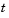.

The PDEs are

•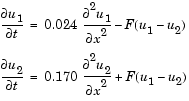where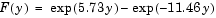. The equations hold on an interval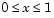for times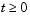.

The solution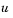satisfies the initial conditions

•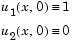and boundary conditions

•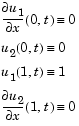Note    The demo `pdex4` contains the complete code for this example. The demo uses subfunctions to place all required functions in a single M-file. To run this example type `pdex4` at the command line. See PDE Solver Basic Syntax and Solving PDE Problems for more information.

1. Rewrite the PDE. In the form expected by `pdepe`, the equations are
•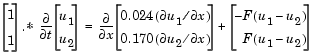1. The boundary conditions on the partial derivatives ofhave to be written in terms of the flux. In the form expected by `pdepe`, the left boundary condition is

•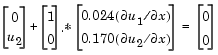and the right boundary condition is

•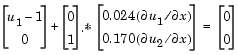1. Code the PDE. After you rewrite the PDE in the form shown above, you can code it as a function that `pdepe` can use. The function must be of the form
• ``````[c,f,s] = pdefun(x,t,u,dudx)
``````
1. where `c`, `f`, and `s` correspond to the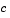,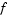, and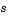terms in Equation 5-3.

• ```function [c,f,s] = pdex4pde(x,t,u,DuDx)
c = [1; 1];
f = [0.024; 0.17] .* DuDx;
y = u(1) - u(2);
F = exp(5.73*y)-exp(-11.47*y);
s = [-F; F];
```
1. Code the initial conditions function. The initial conditions function must be of the form
• ``````u = icfun(x)
``````
1. The code below represents the initial conditions in the function `pdex4ic`.

• ```function u0 = pdex4ic(x);
u0 = [1; 0];
```
1. Code the boundary conditions function. The boundary conditions functions must be of the form
• ``````[pl,ql,pr,qr] = bcfun(xl,ul,xr,ur,t)
``````
1. The code below evaluates the components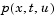and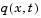(Equation 5-5) of the boundary conditions in the function `pdex4bc`.

• ```function [pl,ql,pr,qr] = pdex4bc(xl,ul,xr,ur,t)
pl = [0; ul(2)];
ql = [1; 0];
pr = [ur(1)-1; 0];
qr = [0; 1];
```
1. Select mesh points for the solution. The solution changes rapidly for small. The program selects the step size in time to resolve this sharp change, but to see this behavior in the plots, output times must be selected accordingly. There are boundary layers in the solution at both ends of [0,1], so mesh points must be placed there to resolve these sharp changes. Often some experimentation is needed to select the mesh that reveals the behavior of the solution.
• ```x = [0 0.005 0.01 0.05 0.1 0.2 0.5 0.7 0.9 0.95 0.99 0.995 1];
t = [0 0.005 0.01 0.05 0.1 0.5 1 1.5 2];
```
2. Apply the PDE solver. The example calls `pdepe` with `m = 0`, the functions pdex4pde, pdex4ic, and pdex4bc, and the mesh defined by x and t at which `pdepe` is to evaluate the solution. The `pdepe` function returns the numerical solution in a three-dimensional array `sol`, where `sol(i,j,k)` approximates the `k`th component of the solution,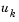, evaluated at `t(i)` and `x(j)`.
• ```m = 0;
sol = pdepe(m,@pdex4pde,@pdex4ic,@pdex4bc,x,t);
```
3. View the results. The surface plots show the behavior of the solution components.
• ```u1 = sol(:,:,1);
u2 = sol(:,:,2);

figure
surf(x,t,u1)
title('u1(x,t)')
xlabel('Distance x')
ylabel('Time t')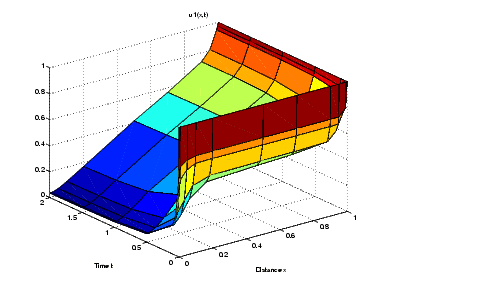figure
surf(x,t,u2)
title('u2(x,t)')
xlabel('Distance x')
ylabel('Time t')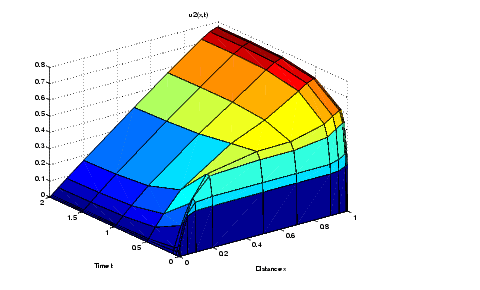```Evaluating the Solution at Specific Points Selected Bibliography© 1994-2005 The MathWorks, Inc.